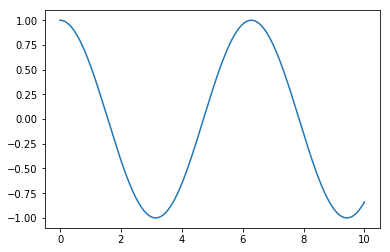# Notebook for Github Test¶

In [ ]:


In :
import numpy as np
import matplotlib.pyplot as plt

a = np.linspace(0,10,1000)
b = np.cos(a)

plt.plot(a,b)

Out:
[<matplotlib.lines.Line2D at 0x7f57bb7164d0>]In :
import heapq
a = []
b = []

heapq.heappush(a,5)
heapq.heappush(b,a)
print(a)
print(b)


[]